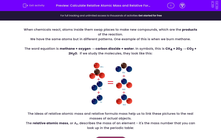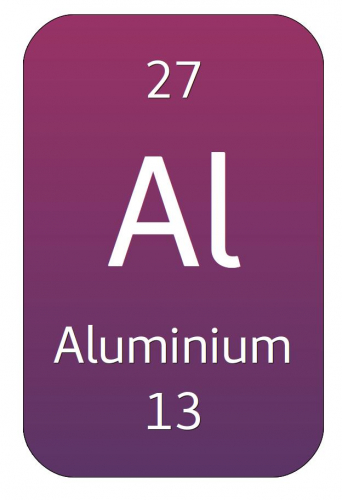# Calculate Relative Atomic Mass and Relative Formula Mass

In this worksheet, students will learn the meaning of relative formula mass, how to calculate it and how to use it to predict the amount of chemical made in a reaction.Key stage:  KS 4

Year:  GCSE

GCSE Boards:   AQA, AQA Trilogy, AQA Synergy, OCR 21st Century, OCR Gateway, Pearson Edexcel, Eduqas,

Difficulty level:#### Worksheet Overview

When chemicals react, atoms inside them swap places to make new compounds, which are the products of the reaction.

We have the same atoms but in different patterns. One example of this is when we burn methane.

The word equation is methane + oxygen → carbon dioxide + water. In symbols, this is CH4 + 2O2 → CO2 + 2H2O.  If we study the molecules, they look like this:The ideas of relative atomic mass and relative formula mass help us to link these pictures to the real masses of actual objects.

The relative atomic mass, or Ar, describes the mass of an element - it's the mass number that you can look up in the periodic table:The mass number is the larger of the two numbers - so the mass number of aluminium is 27.

Although we often quote mass numbers as whole numbers, most are not exactly integers. The reason for this is that elements have different isotopes (atoms with the same number of protons, but different numbers of neutrons).

For example, the relative atomic mass of chlorine is 35.5. This is an average taken from most chlorine atoms having a mass of 35 (called chlorine-35), and some having a mass of 37 (called chlorine-37).

Originally, the relative atomic mass scale was based on hydrogen having one unit. Later on, it was found that using carbon as the basis of the relative atomic mass scale gave better results. Now one unit of relative atomic mass is one-twelfth of the mass of a carbon-12 atom.

To work out the relative formula mass (Mr) of a compound or molecule, we just add the relative atomic masses of all the atoms in a chemical formula. Be careful to take account of the number of copies of each atom in the formula.

For example:

What is the relative formula mass of carbon dioxide, CO2?1. Look up Ar for each of the atoms:   C = 12, O = 16.

2. Add up the relative atomic masses, counting the numbers of each atom. Mr = 12 + (2 x 16) = 44.

It's a good idea to put brackets round the multiplications for each element - this makes sure that you get the right answer.

Some chemical formulas have brackets - copper nitrate is Cu(NO3)2. This means there are two units of NO3 in the formula. The atom count for this formula is CuN2O6.

The reason that relative formula masses are so useful is that they link atomic-scale and real world conservation of mass.

Let's go back to the burning (or combustion) or methane:

The relative formula masses are CH4: 12 + (4 x 1) = 16.  O2 = (2 x 16) = 32. CO2 = 12 + (2 x 16) = 44. H2O = (2 x 1) + 16 = 18.

The total relative formula mass on the reactants side is 16 + (2 x 32) = 80. (We've doubled the mass of O2 because there are two O2's in the reaction).

The total relative formula mass on the products side is 44 + (2 x 18) = 80.

The clever thing is that the relative formula masses work whatever units we use. So 80 g of reactant make 80 g of product; 80 tonnes of reactant make 80 tonnes of product.

They also show balances between individual chemicals: 16 g of methane needs to react with 64 g of oxygen, and they make 44 g of carbon dioxide and 36 g of water.

Relative formula masses are a really powerful tool for predicting amounts of chemical produced in reactions. Like any calculations, don't take short cuts, so you don't get confused.

Time for some questions now.

### What is EdPlace?

We're your National Curriculum aligned online education content provider helping each child succeed in English, maths and science from year 1 to GCSE. With an EdPlace account you’ll be able to track and measure progress, helping each child achieve their best. We build confidence and attainment by personalising each child’s learning at a level that suits them.

Get started